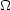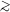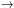4.2. Solving the Flatness Problem

How does inflation solve this flatness problem? How does inflation set up the condition of= 1? Consider Eq. 14: (1 -)H2 R2 = constant. During inflation H = (inf / 3)1/2 = constant (Eq. 22) and the scale factor R increases by many orders of magnitude,1030. One can then see from Eq. 14 that the large increase in the scale factor R during inflation, with H constant, drives1. This is what is meant when we say that inflation makes the Universe spatially flat. In a vacuum-dominated expanding universe,= 1 is a stable fixed point. During inflation H is constant and R increases exponentially. Thus, no matter how faris from 1 before inflation, the exponential increase of R during inflation quickly drives it to 1 and this is equivalent to flattening the Universe. Once driven to= 1 by inflation, the Universe will naturally evolve away from= 1 in the absence of inflation as we showed in the previous section.